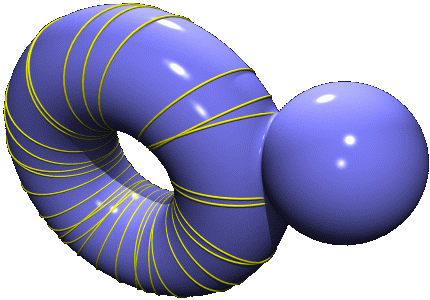Titel (html) Vorwort (html) Inhalt (html) Title (html) Preface (html) Content (html)

The finished version is available as a book in the series "Lectures in Mathematics ETH Zürich".

 Review by V. Bangert, Jan 2005

 Known errata: Theorem 1.4.4. Let $\gamma^*$ be an extremal with no conjugate points. Then $\gamma^*$ can be embedded in an extremal field. (Translation error). Theorem 3.6.1. ... there are at least dim(H_1(M,R)) minimal geodesics (Typo parenthesis). Section 1.5: In invariant terminology, an n-dimensional submanifold (comma missing)Gallerie von Illustrationen

 © 1999-2002, www.dynamical-systems.org